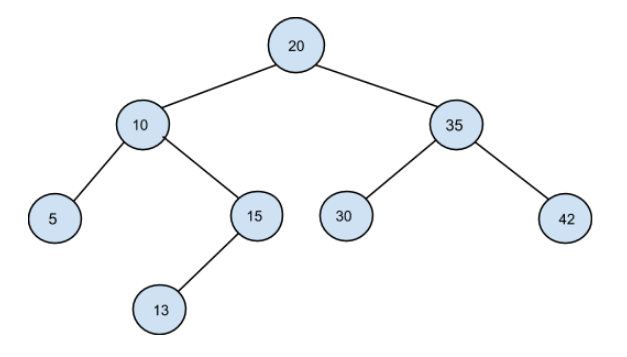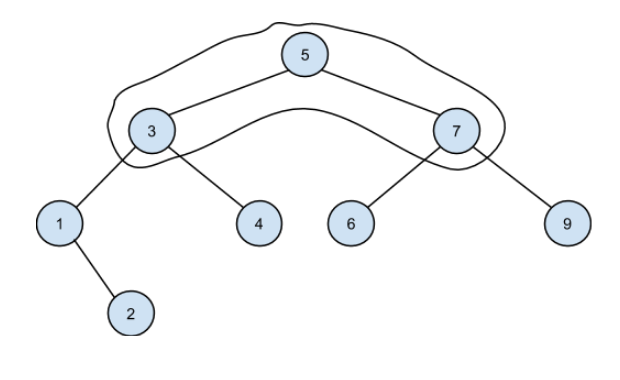9

# Max element in the path

Difficulty: EASYContributed By
Sounak Majumder
Avg. time to solve
15 min
Success Rate
85%

Problem Statement
Suggest Edit

#### Note:

``````1. The path from NODE1 to NODE2 does not include NODE1 and NODE2.

2. NODE1 and NODE2 are unique.

3. If there is no element in the path from NODE1 to NODE2, return -1.
``````
##### Input format:
``````The first line of input contains an integer ‘T’ denoting the number of test cases.

Each test case follows:
The first line of every test case contains elements in the level order form. The input consists of values of nodes separated by a single space in a single line. In case a node is null, we take -1 in its place.

The second line contains two space-separated integers denoting NODE1 and NODE2.

For example, the input for the tree depicted in the below image would be :
````````````20
10 35
5 15 30 42
-1 13 -1 -1 -1 -1 -1
-1 -1

Explanation:

Level 1 :
The root node of the tree is 20
Level 2 :
Left child of 20 = 10
Right child of 20 = 35
Level 3 :
Left child of 10 = 5
Right child of 10 = 15
Left child of 35 = 30
Right child of 35 = 42
Level 4 :
Left child of 5 = null (-1)
Right child of 5 = null (-1)
Left child of 15 = 13
Right child of 15 = null (-1)
Left child of 30 = null (-1)
Right child of 30 = null (-1)
Left child of 42 = null (-1)
Right child of 42 = null (-1)
Level 5 :
Left child of 13 = null (-1)
Right child of 13 = null (-1)

The first not-null node (of the previous level) is treated as the parent of the first two nodes of the current level. The second not-null node (of the previous level) is treated as the parent node for the next two nodes of the current level and so on.

The input ends when all nodes at the last level are null (-1).
``````

#### Note:

``````The above format was just to provide clarity on how the input is formed for a given tree.

The sequence will be put together in a single line separated by a single space. Hence, for the above-depicted tree, the input will be given as:
20 10 35 5 15 30 42 -1 13 -1 -1 -1 -1 -1 -1 -1
``````
##### Output Format
``````For each test case, print a single line containing a single integer denoting the maximum element in the path from NODE1 to NODE2. If either NODE1 or NODE2 does not exist in the BST, return -1.

The output of each test case will be printed in a separate line.
``````
##### Note
``````You don’t have to print anything; it has already been taken care of. Just complete the function.
``````
##### Constraints
``````1 <= T <= 100
1 <= N <= 3000
0 <= DATA <= 10 ^ 9
1 <= NODE1, NODE2 <= 10 ^ 9

Where ‘T’ is the total number of test cases, 'N' denotes the number of nodes in the given BST,  'DATA' denotes the value of each node in the given tree, and NODE1 and NODE2 are 2 integers.

Time limit: 1 sec.
``````
##### Sample Input 1:
``````1
5 3 7 1 4 6 9 -1 2 -1 -1 -1 -1 -1 -1 -1 -1
1 9
``````
##### Sample Output 1:
``````7
``````
##### Explanation of sample input 1:
``````The BST of the given elements is shown below.
````````````The path from 1 to 9 is shown in the curve.
As we can see, the maximum element in the path from 1 to 9 is 7.
``````
##### Sample Input 2:
``````2
2 1 4 -1 -1 3 -1 -1 -1
3 5
7 5 8 -1 6 -1 10 -1 -1 9 -1 -1 -1
6 7
``````
##### Sample Output 2:
``````-1
5
``````
##### Explanation of sample input 2:
``````Test case 1:
As 5 does not exist in the BST, simply return -1.

Test case 2:
After creating the BST for the given level order, we can see that the 5 is the maximum element between 6 and 7.
``````Console